NEET  >  Test: Crystal Field Theory (CFT) & Colour of Complexes

# Test: Crystal Field Theory (CFT) & Colour of Complexes

Test Description

## 25 Questions MCQ Test Chemistry Class 12 | Test: Crystal Field Theory (CFT) & Colour of Complexes

Test: Crystal Field Theory (CFT) & Colour of Complexes for NEET 2023 is part of Chemistry Class 12 preparation. The Test: Crystal Field Theory (CFT) & Colour of Complexes questions and answers have been prepared according to the NEET exam syllabus.The Test: Crystal Field Theory (CFT) & Colour of Complexes MCQs are made for NEET 2023 Exam. Find important definitions, questions, notes, meanings, examples, exercises, MCQs and online tests for Test: Crystal Field Theory (CFT) & Colour of Complexes below.
Solutions of Test: Crystal Field Theory (CFT) & Colour of Complexes questions in English are available as part of our Chemistry Class 12 for NEET & Test: Crystal Field Theory (CFT) & Colour of Complexes solutions in Hindi for Chemistry Class 12 course. Download more important topics, notes, lectures and mock test series for NEET Exam by signing up for free. Attempt Test: Crystal Field Theory (CFT) & Colour of Complexes | 25 questions in 35 minutes | Mock test for NEET preparation | Free important questions MCQ to study Chemistry Class 12 for NEET Exam | Download free PDF with solutions
 1 Crore+ students have signed up on EduRev. Have you?
Test: Crystal Field Theory (CFT) & Colour of Complexes - Question 1

### Direction (Q. Nos. 1-10) This section contains 10 multiple choice questions. Each question has four choices (a), (b), (c) and (d), out of which ONLY ONE is correct. Q.  Among the following complexes the one which shows zero crystal field stabilisation energy is

Detailed Solution for Test: Crystal Field Theory (CFT) & Colour of Complexes - Question 1

In [Fe(H2O)6]3+, iron has 3d5 configuration. Since, H2O is weak ligand the distribution is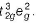.
Hence, CFSE = - 3 x 0.4 + 2 x 0.6 = 0.0

Test: Crystal Field Theory (CFT) & Colour of Complexes - Question 2

### The wavelength of light absorbed is highest in

Detailed Solution for Test: Crystal Field Theory (CFT) & Colour of Complexes - Question 2

Among all the coordination aforesaid in complex [Co(NH3)5Cl]2+ unpaired electrons are present with the central element cobalt so it required less energy for excitation. Thus, the wavlength of light absorbed will be the highest.

Test: Crystal Field Theory (CFT) & Colour of Complexes - Question 3

### The CFSE for octahedral [CoCI6]4- is 18000 cm-1. Then the CFSE for tetrahedral [CoCI4]2- will be

Detailed Solution for Test: Crystal Field Theory (CFT) & Colour of Complexes - Question 3

CFSE for [CoCI4]2- will be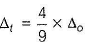i.e.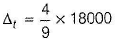= 8000cm-1

Test: Crystal Field Theory (CFT) & Colour of Complexes - Question 4

The crystal field splitting energy (Δo) of

I. [CoBr6]3- II. [CoF6]3-
III. [Co(NCS)6]3- IV. [Co(CN)6]3- is in the order of

Detailed Solution for Test: Crystal Field Theory (CFT) & Colour of Complexes - Question 4

Crystal field splitting energy depends on strength of ligand. Strong ligands have more value of CFSE (Δo).
Hence, Br- < F- < NCS < CN-

Test: Crystal Field Theory (CFT) & Colour of Complexes - Question 5

A magnetic moment of 1.73 BM will be shown by one among the following

Detailed Solution for Test: Crystal Field Theory (CFT) & Colour of Complexes - Question 5

The correct answer is Option A.
Electronic configuration of Cu2+  ion in [Cu(NH3)4]2+.
Cu2+ ion =[Ar]3d94s0.
∴Cu2+ ion has one unpaired electron.
Magnetic moment of [Cu(NH3)4]2+ (μ) =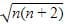BM
where, n = no. of unpaired electrons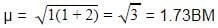Whereas Ni2+ in [Ni(CN)4]2− , Ti4+ in TiCl4 and Co2+ ion [COCl6]4− has 2,0 and 3 unpaired electrons respectively.

Test: Crystal Field Theory (CFT) & Colour of Complexes - Question 6

The correct order o f ligand field strength is

Test: Crystal Field Theory (CFT) & Colour of Complexes - Question 7

The crystal field splitting energies (CFSE) of high spin and low spin d6 metal complexes in octahedral complex in terms of Δo respectively are

Detailed Solution for Test: Crystal Field Theory (CFT) & Colour of Complexes - Question 7

For high spin d6, CFSE = - 4 x 0.4 + 2 x 0.6 = -0.4
For low spin d6, CFSE= - 6 x 0.4 = -2.4

Test: Crystal Field Theory (CFT) & Colour of Complexes - Question 8

The Δt of the following complexes follows the order

I. [CoCI4]2-

II. [CoBr4]2-

III. [Co(NCS)4]2-

Detailed Solution for Test: Crystal Field Theory (CFT) & Colour of Complexes - Question 8

Explanation : Bonding Energy :

[CoCI4]2      -2084.67

[CoBr4]2      -3139.39

[Co(NCS)4]2-  - 8559.48

Correct order is : III > II > I

Test: Crystal Field Theory (CFT) & Colour of Complexes - Question 9

In which of the following coordination entities, the magnitude Δo (CFSE in octahedral field) will be maximum ?

Detailed Solution for Test: Crystal Field Theory (CFT) & Colour of Complexes - Question 9

Increasing order of ligand field strength
l- < Br- < SCN- < CI- < S2- < F- < OH-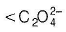< H2O < NCS- < edta4- < NH3 < en < CN- < CO

Test: Crystal Field Theory (CFT) & Colour of Complexes - Question 10

The increasing order of wavelength of absorption for the complex ions

I. [Cr(NH3)6]3+

II. [CrCI6]3-

III. [Cr(H2O)6]3+
IV. [Cr(CN)6]3-

is :

Detailed Solution for Test: Crystal Field Theory (CFT) & Colour of Complexes - Question 10

Stronger ligand causes greater splitting between two energy levels.

The energy of light absorbed is more and the wavelength of light absorbed is less for the stronger ligand.

Hence order of wavelength absorption will be reverse of the order of the strength of ligands.

Hence A is correct.

*Multiple options can be correct
Test: Crystal Field Theory (CFT) & Colour of Complexes - Question 11

Direction (Q. Nos. 11-15) This section contains 5 multiple choice questions. Each question has four choices (a), (b), (c) and (d), out of which ONE or MORE THAN ONE are correct.

Q.

Which of the following metal ions cannot form both high spin and low spin octahedral complexes?

Detailed Solution for Test: Crystal Field Theory (CFT) & Colour of Complexes - Question 11

Ti3+ has 3d1 configuration and Cu2+ has 3d9 configuration.

*Multiple options can be correct
Test: Crystal Field Theory (CFT) & Colour of Complexes - Question 12

Which is/are the correct statements?

Detailed Solution for Test: Crystal Field Theory (CFT) & Colour of Complexes - Question 12

If Δo < P high spin complexes are favoured.

*Multiple options can be correct
Test: Crystal Field Theory (CFT) & Colour of Complexes - Question 13

CFSE is zero for the complexes with

Detailed Solution for Test: Crystal Field Theory (CFT) & Colour of Complexes - Question 13

For d5 in weak field, CFSE = -3 x 0.4 + 2 x 0.6 = 0
For d10 in weak and strong ligand field, CFSE = -6 x 0.4 + 4 x 0.6 = 0

*Multiple options can be correct
Test: Crystal Field Theory (CFT) & Colour of Complexes - Question 14

CFSE value depends on

Detailed Solution for Test: Crystal Field Theory (CFT) & Colour of Complexes - Question 14

Geometry of complex, charge on metal ion, nature of ligands and series of d orbital are the factors on which CFSE value depends.

*Multiple options can be correct
Test: Crystal Field Theory (CFT) & Colour of Complexes - Question 15

Octahedral complex of Cr (III) will be

Detailed Solution for Test: Crystal Field Theory (CFT) & Colour of Complexes - Question 15

Cr (III) ion have 3d3 configuration.
Cr (III) ion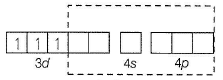Flence, it will form always d2sp3 hybridisation and show colour due to the presence of 3 unpaired electrons.

Test: Crystal Field Theory (CFT) & Colour of Complexes - Question 16

Comprehension Type

Direction (Q. Nos. 16 and 17) This section contains a paragraph, describing theory, experiments, data, etc. Two questions related to the paragraph have.

Passage

In octahedral complexes due to repulsion between the ligands and d-orbitals, there is splitting of d-orbitals into two sets, i.e. two orbitals of higher energy called eg and three orbitals of lower energy called t2g. The difference of energy between the two sets of d-orbitals is called crystal field stabilisation energy denoted by Δo. For any given metal cation, the magnitude of Δo depends on the nature of ligands.

Q.

The CFSE for d7 configuration for strong ligand field is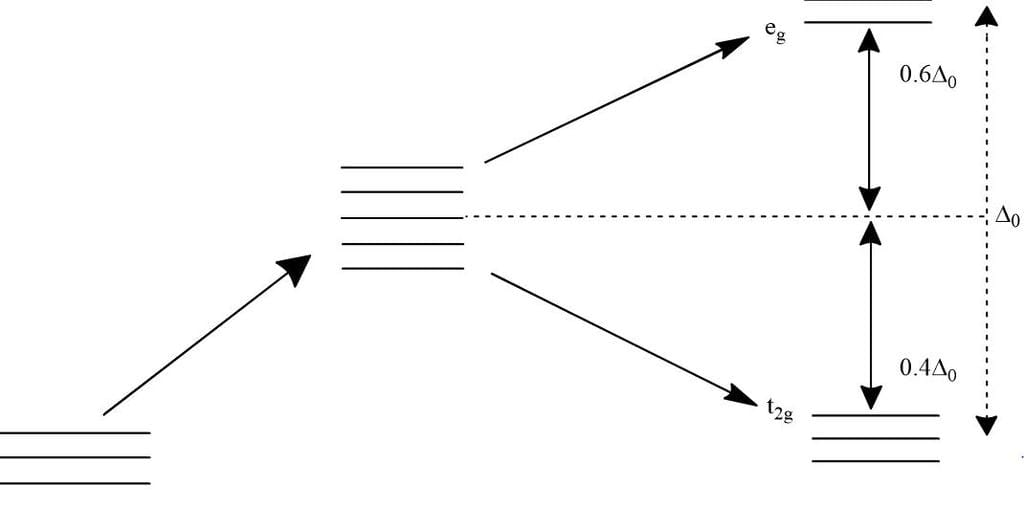Detailed Solution for Test: Crystal Field Theory (CFT) & Colour of Complexes - Question 16

The total crystal field stabilisation energy is given by CFSE(octahedral) =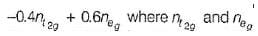are the number of electron occupying the t2g and eg orbitatls respectively.
For d7 in strong field,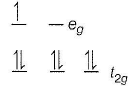CFSE = -0.4 x 6 + 0.6 x 1 = -2.4 + 0.6 = -1.8Δo

Test: Crystal Field Theory (CFT) & Colour of Complexes - Question 17

In octahedral complexes due to repulsion between the ligands and d-orbitals, there is splitting of d-orbitals into two sets, i.e. two orbitals of higher energy called eg and three orbitals of lower energy called t2g. The difference of energy between the two sets of d-orbitals is called crystal field stabilisation energy denoted by Δo. For any given metal cation, the magnitude of Δo depends on the nature of ligands.

Q.

In the following complexes of manganese, the distribution of electrons in d-orbitals of manganese

i. [Mn(H2O)6]2+

ii. [Mn(CN)6]4-

Detailed Solution for Test: Crystal Field Theory (CFT) & Colour of Complexes - Question 17

In [Mn (H2O)6]2+, manganese have d5 configuration. H2O is a weak field ligand. Hence, it will form high spin complex.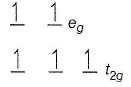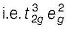In [Mn(CN)6]4-, manganese have d5 configuration. CN- ion is a strong field ligand. It will form low spin complex by pairing of electron in t2g orbitals.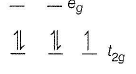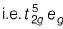Test: Crystal Field Theory (CFT) & Colour of Complexes - Question 18

Matching List Type

Direction (Q. No. 18-19) Choices for the correct combination of elements from Column I and Column II are given as options (a), (b), (c) and (d) out of which one is correct.

Q.

Match the Column I with Column II and mark the correct option from the codes given below.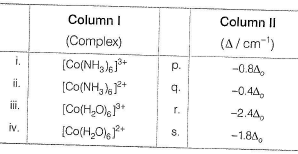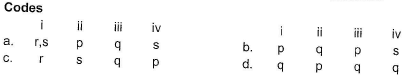Detailed Solution for Test: Crystal Field Theory (CFT) & Colour of Complexes - Question 18

(i) → (r), (ii) → (s), (iii) → (q), (iv) → (p)

Test: Crystal Field Theory (CFT) & Colour of Complexes - Question 19

Match the Column I with Column II and mark the correct option from the codes given below.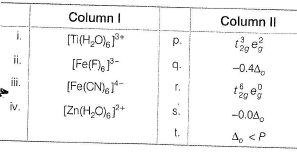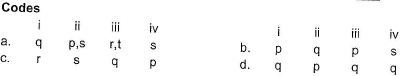Detailed Solution for Test: Crystal Field Theory (CFT) & Colour of Complexes - Question 19

(i) → (q), (ii) → (p,s), (iii) → (r,t), (iv) → (s)

*Answer can only contain numeric values
Test: Crystal Field Theory (CFT) & Colour of Complexes - Question 20

One Integer Value Correct Type

Direction (Q. Nos. 20-24) This section contains 5 questions. When worked out will result in an integer from 0 to 9 (both inclusive).

Q.

Number of unpaired electrons in d6, low spin octahedral complex.

Detailed Solution for Test: Crystal Field Theory (CFT) & Colour of Complexes - Question 20

In d6 low spin octahedral complex.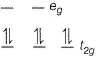Hence, zero number of unpaired electrons.

*Answer can only contain numeric values
Test: Crystal Field Theory (CFT) & Colour of Complexes - Question 21

The number of ligands which have strong crystal field splitting than

H2O among SCN-, NCS-, EDTA4- ,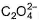,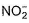, Br-, PPh3, F-

Detailed Solution for Test: Crystal Field Theory (CFT) & Colour of Complexes - Question 21

NCS- edta4- ,and PPh3 are strong field ligand than H2O.

*Answer can only contain numeric values
Test: Crystal Field Theory (CFT) & Colour of Complexes - Question 22

The total number of unpaired electrons in the two complexes [Cr(H2O)6]2+ and [Cr(CN)6]4- having octahedral geometry are

Detailed Solution for Test: Crystal Field Theory (CFT) & Colour of Complexes - Question 22

In [Cr(H2O)6]2+ chromium have d4 configuration and H2O is a weak field ligand.
d4 (weak field)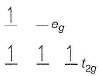In [Cr(CN)6]4- chromium have d4 configuration and CN- ion is a strong field ligand. Hence, CN- ion causing pairing of electron. d4 (strong field)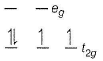Hence, total number of unpaired electrons in both the complex are 6.

*Answer can only contain numeric values
Test: Crystal Field Theory (CFT) & Colour of Complexes - Question 23

Number of unpaired electrons in t2g and eg orbitals in weak octahedral ligand fields with d7 configuration.

Detailed Solution for Test: Crystal Field Theory (CFT) & Colour of Complexes - Question 23

d7 (weak octahedral field)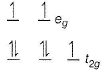Hence, 3 unpaired electrons.

*Answer can only contain numeric values
Test: Crystal Field Theory (CFT) & Colour of Complexes - Question 24

In tetrahedral complexes having weak field ligand, pairing starts with ...... electron.

Detailed Solution for Test: Crystal Field Theory (CFT) & Colour of Complexes - Question 24

The pairing starts with six electrons in the tetrahedral complexes which have weak field ligand.

Test: Crystal Field Theory (CFT) & Colour of Complexes - Question 25

Statement Type

Direction (Q. No. 25) This section is based on Statement I and Statement II. Select the correct answer from the codes given below.

Q.

Statement I : The value of CFSE for the octahedral complex [Co(NH3)6]3+ is - 2.4Δo.

Statement II : The ammonia molecule is strong field ligand.

Detailed Solution for Test: Crystal Field Theory (CFT) & Colour of Complexes - Question 25

In [Co(NH3)6]3+ Co, have d6 configuration and NH3 is strong field ligand.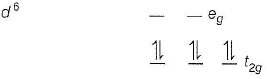CFSE(OCt) = -0.4 x 6 + 0.6 x 0 = -2.4Δo

## Chemistry Class 12

157 videos|385 docs|261 tests
 Use Code STAYHOME200 and get INR 200 additional OFF Use Coupon Code
Information about Test: Crystal Field Theory (CFT) & Colour of Complexes Page
In this test you can find the Exam questions for Test: Crystal Field Theory (CFT) & Colour of Complexes solved & explained in the simplest way possible. Besides giving Questions and answers for Test: Crystal Field Theory (CFT) & Colour of Complexes, EduRev gives you an ample number of Online tests for practice

## Chemistry Class 12

157 videos|385 docs|261 tests

### How to Prepare for NEET

Read our guide to prepare for NEET which is created by Toppers & the best Teachers